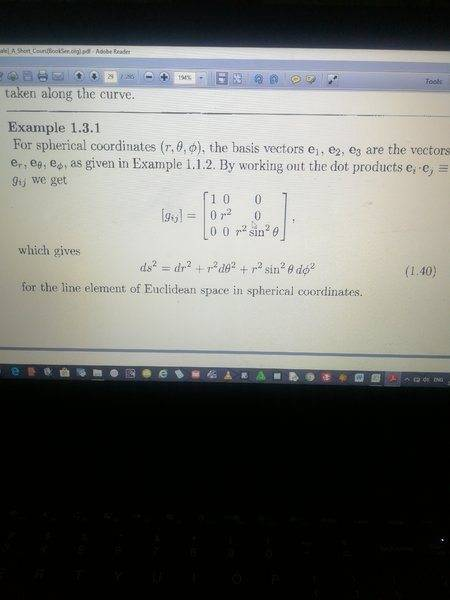# How was the matrix in the attachment found

• A
LSMOGI understand the dot product of ei.ej, but I can't find the matrix components.

#### Attachments

I understand the dot product of ei.ej, but I can't find the matrix components.
The matrix components are these dot products.

LSMOG
Thanks. I've managed to find the matrix above by finding the dot products. Now how was ds2 = dr2+r22+r2 sin2θ dΦ2 found?

Homework Helper
Gold Member
sweet springs
You can get it by inserting
$$x=r\ sin\theta\ cos\phi$$
$$y=r\ sin\theta\ sin\phi$$
$$z=r\ cos\ \theta$$
into
$$dx^2+dy^2+dz^2$$

LSMOG
You can get it by inserting
$$x=r\ sin\theta\ cos\phi$$
$$y=r\ sin\theta\ sin\phi$$
$$z=r\ cos\ \theta$$
into
$$dx^2+dy^2+dz^2$$
Thank you. But you you gave x, y and z in spherical coordinates, according to your last expression, I need dx, dy and dx in spherical coordinates.